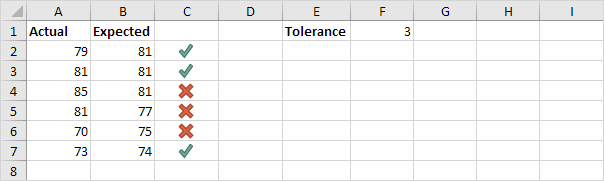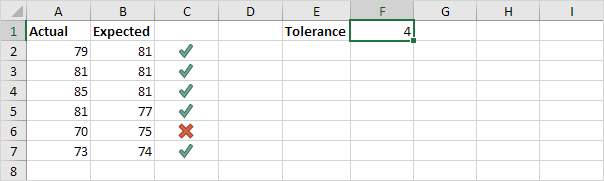# Absolute Value

The ABS function in Excel returns the absolute value of a number. In other words: the ABS function removes the minus sign (-) from a negative number, making it positive.

1. For example, the ABS function in cell B1 below returns the absolute value of a negative number.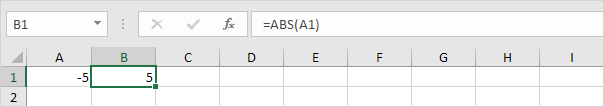The ABS function has no effect on 0 (zero) or positive numbers.

2. The absolute value of 0 is 0.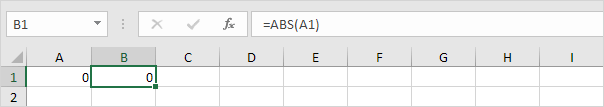3. The absolute value of a positive number is the same positive number.When do we need the ABS function in Excel?

4. For example, calculate the forecast error (difference between the actual and the forecast value) for each month.Note: if we look at the sum of these errors (zero), this forecast model seems perfect, but it's not!

5. Simply use the ABS function to calculate the absolute error for each month.6. If you don't want to display the forecast errors on the sheet, use SUMPRODUCT and ABS.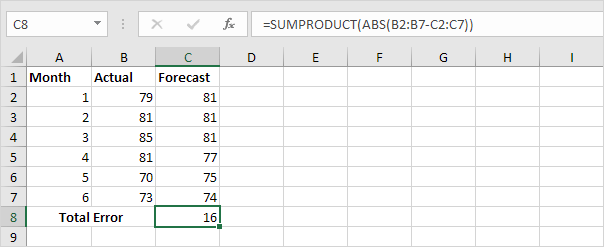Let's take a look at one more cool example.

7. Use the ABS function to calculate the absolute value of each difference.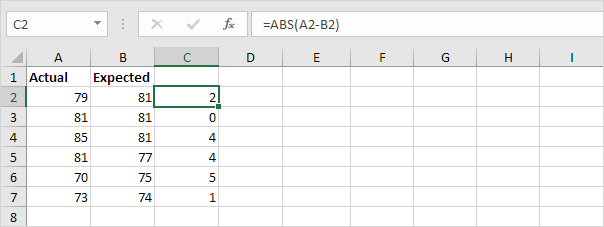8. Add the IF function to test if the values are within tolerance.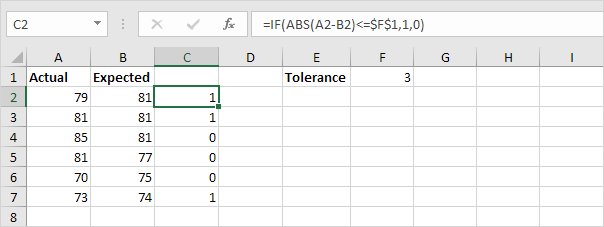Explanation: if the difference is less than or equal to 3, the IF function returns 1, else it returns 0.